# Complementary Angles

Complementary Angles
Go back to  'Angles'

 1 What are Complementary Angles? 2 Adjacent and Non-Adjacent Complementary Angles (With Illustrations) 3 How to Find Complement of an Angle? (With an Activity) 4 Differences between Complementary and Supplementary Angles 5 Tips and Tricks 6 Complementary Angle Theorem (with Illustration) 7 Solved Examples on Complementary Angles 8 Challenging Questions on Complementary Angles 9 Practice Questions on Complementary Angles 10 Maths Olympiad Sample Papers 11 Frequently Asked Questions (FAQs)

We at Cuemath believe that Math is a life skill. Our Math Experts focus on the “Why” behind the “What.” Students can explore from a huge range of interactive worksheets, visuals, simulations, practice tests, and more to understand a concept in depth.

Book a FREE trial class today! and experience Cuemath's LIVE Online Class with your child.

## What are Complementary Angles in Geometry?

### Definition of Complementary Angles

Two angles are said to be complementary angles if they add up to 90 degrees.

In other words, when complementary angles are put together, they form a right angle (90 degrees).

 $$\angle 1$$ and $$\angle 2$$ are complementary if $\angle 1+ \angle 2 = 90^\circ$

In this case, $$\angle 1$$ and $$\angle 2$$ are called complements of each other.

### Examples of Complementary Angles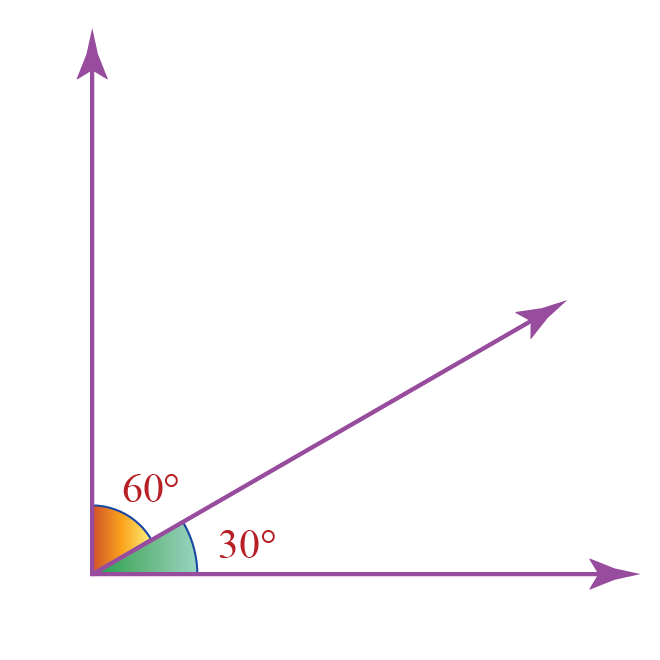Here, $$60^\circ+30^\circ = 90^\circ$$

Hence, from the "Definition of Complementary Angles", these two angles are complementary.

Each angle among the complementary angles is called the "complement" of the other angle.

Here,

• 60o is the complement of 30o
• 30o is the complement of 60o

Some real-life examples of complementary angles are as follows: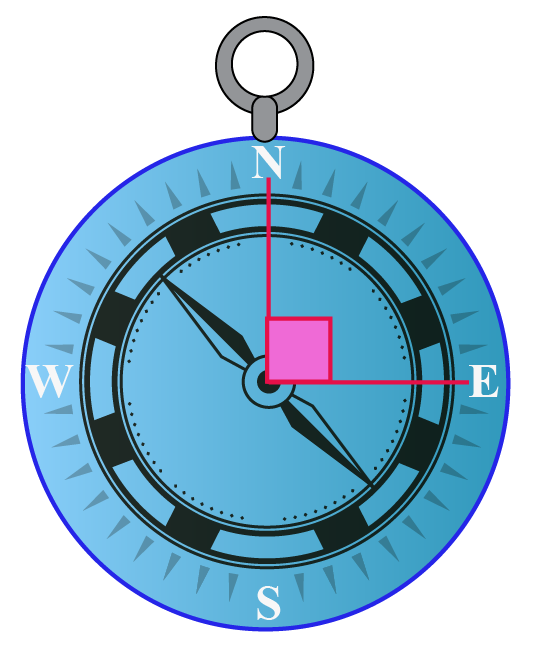The two angles in each of the above figures are adjacent (it means that they have a common vertex and a common arm).

But, do complementary angles always need to be adjacent?

No, they can be non-adjacent as well.

Let us see how.

There are two types of complementary angles in geometry.

Two complementary angles with a common vertex and a common arm are called adjacent complementary angles.

Example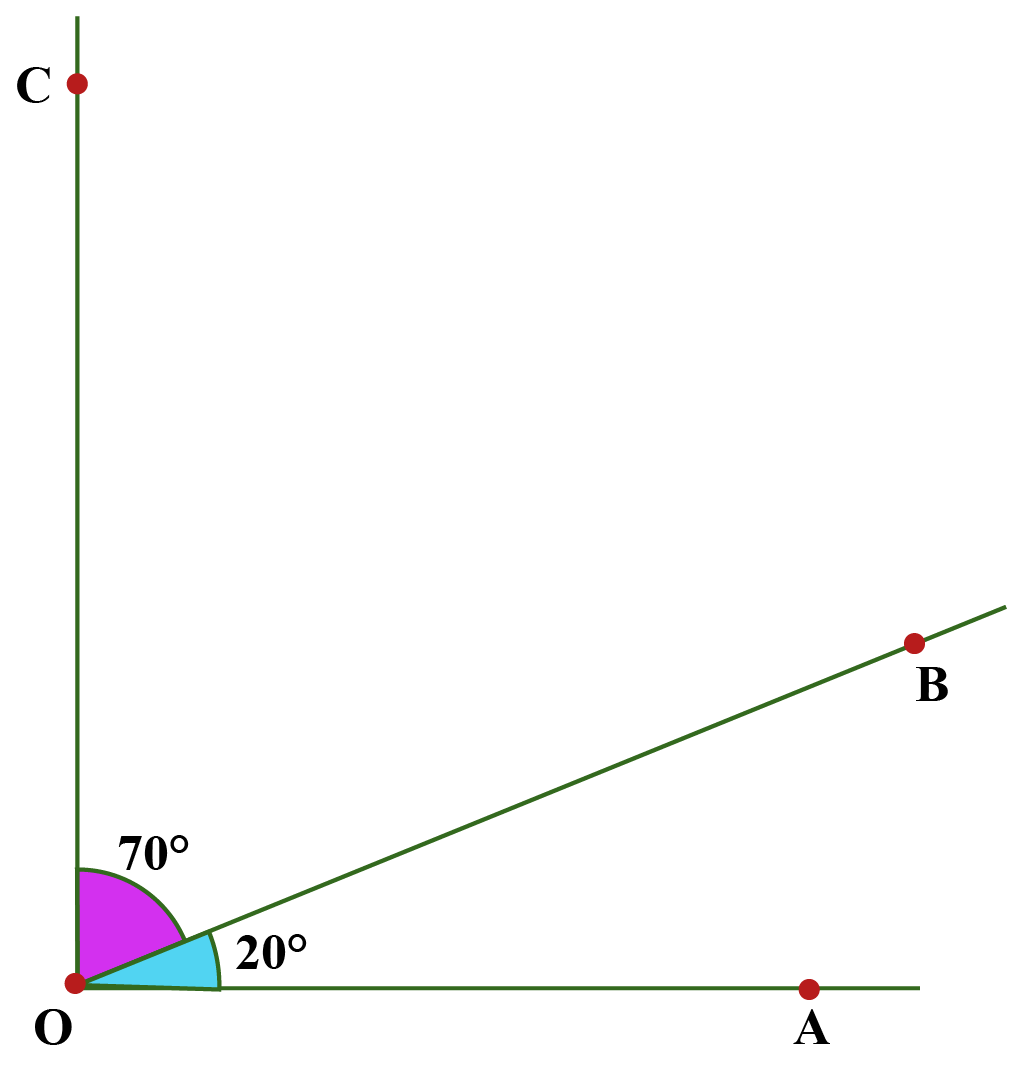Here, $$\angle COB$$ and $$\angle AOB$$ are adjacent angles as they have a common vertex $$O$$ and a common arm $$OB$$.

They also add up to 90 degrees. i.e.,

$\angle COB + \angle AOB = 70^\circ+20^\circ=90^\circ$

Thus, these two angles are adjacent complementary angles.

You can observe adjacent complementary angles using the following illustration.

Move point C to change the angles and click "GO".

Two complementary angles that are NOT adjacent are said to be non-adjacent complementary angles.

Example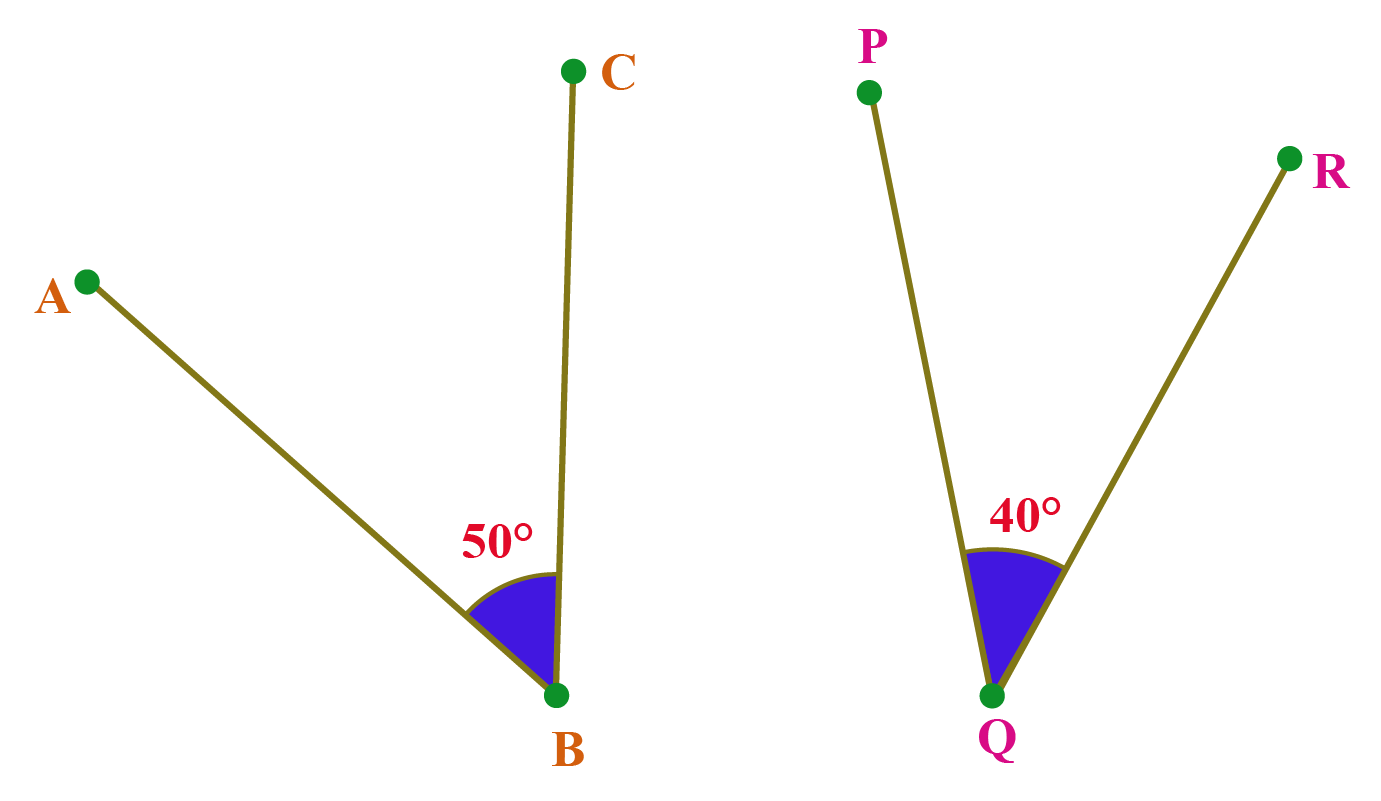Here, $$\angle ABC$$ and $$\angle PQR$$ are non-adjacent angles as they neither have a common vertex nor a common arm.

Also, they add up to 90 degrees. i.e.,

$\angle ABC+ \angle PQR = 50^\circ+40^\circ=90^\circ$

Thus, these two angles are non-adjacent complementary angles.

When non-adjacent complementary angles are put together, they form a right angle.

You can observe this using the following illustration.

Move the first slider to change the angles and move the second slider to see how the angles are complementary.

## How to Find Complement of an Angle? (With an Activity)

We know that the sum of two complementary angles is 90 degrees and each of them is said to be a "complement" of each other.

Thus, the complement of an angle is found by subtracting it from 90 degrees.

 Complement of $$x^\circ$$ is $$(90-x)^\circ$$

Example

What is the complement of 57o?

Solution

The complement of 57o is obtained by subtracting it from 90o

Thus, its complement is:

$(90-57)^\circ =33^\circ$

Here is an activity on "Finding the Complement of an Angle".

You can click and drag the "Orange" dot to change the angles and then you can enter the complement of the given angle.

## Differences between Complementary and Supplementary Angles

Complementary
Angles

Supplementary
Angles

Two angles are said to be complementary if they add up to 90 degrees.

Two angles are said to be supplementary if they add up to 180 degrees.

Complement of an angle $$x^\circ$$ is $$(90-x)^\circ$$

Supplement of an angle $$x^\circ$$ is $$(180-x)^\circ$$Tips and Tricks
1. "S" is for "Supplementary" and "S" is for "Straight".
Hence, you can remember that two "Supplementary" angles when put together form a "Straight" angle.
2. "C" is for "Complementary" and "C" is for "Corner".
Hence, you can remember that two "Complementary" angles when put together form a "Corner (right)" angle.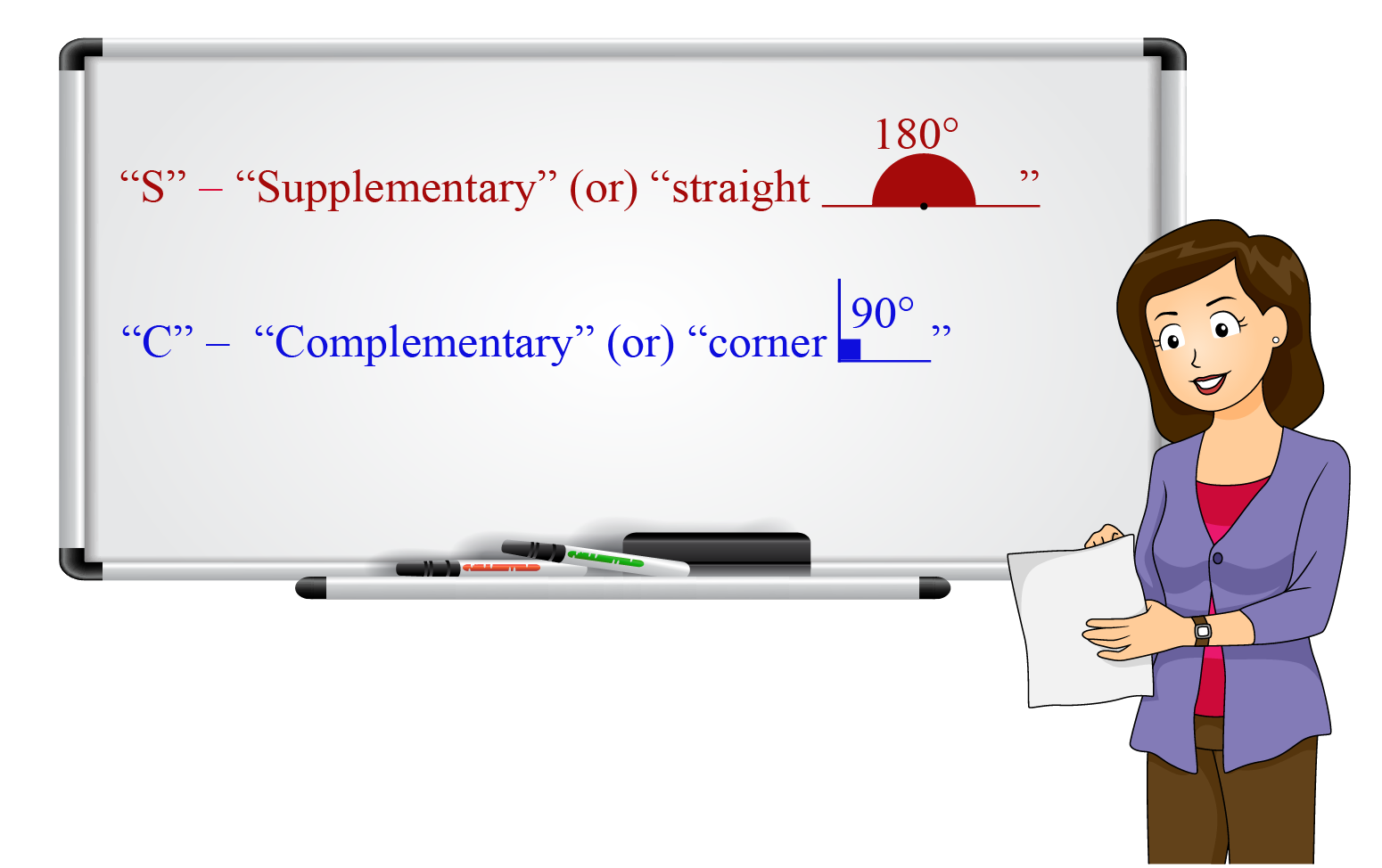## Complementary Angle Theorem (with Illustration)

The complementary angle theorem states, "If two angles are complementary to the same angle, then they are congruent to each other".

### Proof of Complementary Angle Theorem

Consider the following figure: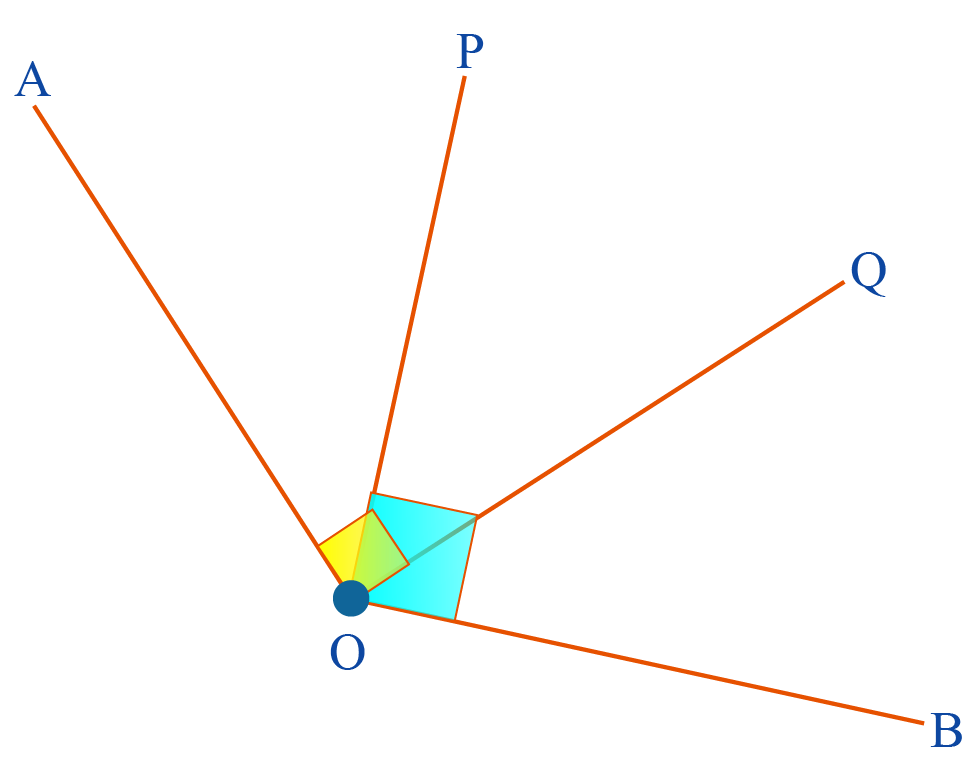Let us assume that $$\angle POQ$$ is complementary to $$\angle AOP$$ and $$\angle BOQ$$.

By the definition of complementary angles,

\begin{align} \angle POQ + \angle AOP &= 90^\circ\\[0.3cm] \angle POQ + \angle BOQ &=90^\circ \end{align} From the above two equations, we can say that $\angle POQ + \angle AOP=\angle POQ + \angle BOQ$ Subtracting $$\angle POQ$$ from both sides, $\angle AOP = \angle BOQ$ Hence, the theorem is proved.

You can visualize the Complementary Angle Theorem using the following illustration.

Help your child score higher with Cuemath’s proprietary FREE Diagnostic Test. Get access to detailed reports, customized learning plans, and a FREE counseling session. Attempt the test now.

## Solved Examples

 Example 1

Find the angle $$x$$ in the following figure.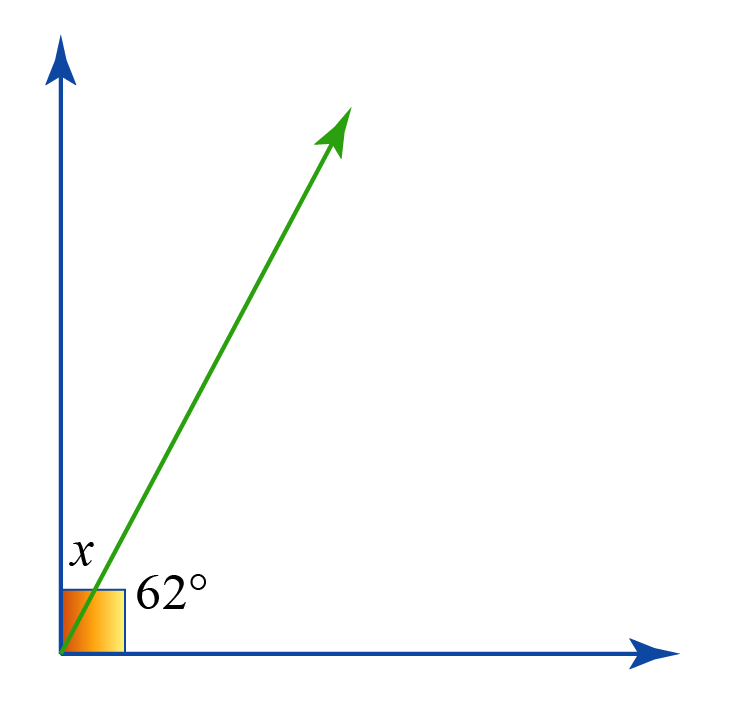Solution:

In the given figure, $$x$$ and 62o are complementary as they form a right angle.

Hence, their sum is 90o

\begin{align} x +62^\circ &= 90^\circ \\[0.2cm] x &= 90^\circ-62^\circ\\[0.2cm] x&= 28^\circ \end{align}

 $$\therefore$$ $$x=28^\circ$$
 Example 2

Find the values of $$\angle A$$ and $$\angle B$$  if $$\angle A$$ and $$\angle B$$ are complementary such that $$\angle A=3x-25$$ and $$\angle B=6x−65$$.

Solution:

Since $$\angle A$$ and $$\angle B$$ are complementary, their sum is 90o.

\begin{align} \angle A+\angle B &=90\\[0.2cm] (3x-25)+(6x-65)&=90\\[0.2cm] 9x - 90&=90\\[0.2cm] 9x&=180\\ x &= 20 \end{align}

Therefore, \begin{align} \angle A &= 3(20)-25 = 35^\circ\\[0.2cm] \angle B &= 6(20)-65 =55^\circ \end{align}

 $$\therefore$$ \begin{align} \angle A &= 35^\circ\0.2cm] \angle B & =55^\circ \end{align}  Example 3 Find the value of $$x$$ if the following two angles are complementary.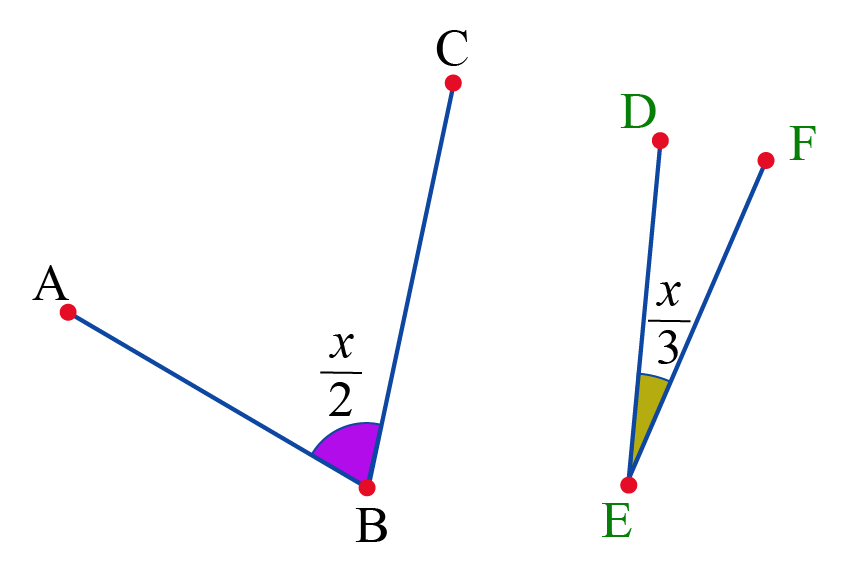Solution: Since the given two angles are complementary, their sum is 90o. \[ \begin{align} \dfrac{x}{2}+ \dfrac{x}{3}&=90\\[0.2cm] \dfrac{5x}{6}&=90\\[0.2cm] x&=90 \times \dfrac{6}{5}\\[0.2cm] x &= 108\end{align}

 $$\therefore$$ $$x = 108$$
 Example 4

Two angles are complementary.

The measure of the larger angle is 5 degrees more than 4 times the measure of the smaller angle.

What is the measure of the larger angle in degrees?

Solution:

Let us assume that the two complementary angles are $$x$$ (larger) and $$y$$ (smaller).

By the given information,$x = 4y+5$

Since the two angles are complementary, their sum is 90o.

\begin{align} x+y&=90\\[0.2cm] (4y+5)+y &=90& [\because x=4y+5]\\[0.2cm] 5y+5&=90\\[0.2cm] 5y&=85\\[0.2cm] y&=17\end{align}

Thus, the larger angle is, $x = 4(17)+5=73^\circ$

 $$\therefore$$ Larger angle = $$73^\circ$$Challenging Questions
1. In the given figure, PQ is perpendicular to PR and ∠QPS=56°. Find ∠RPT.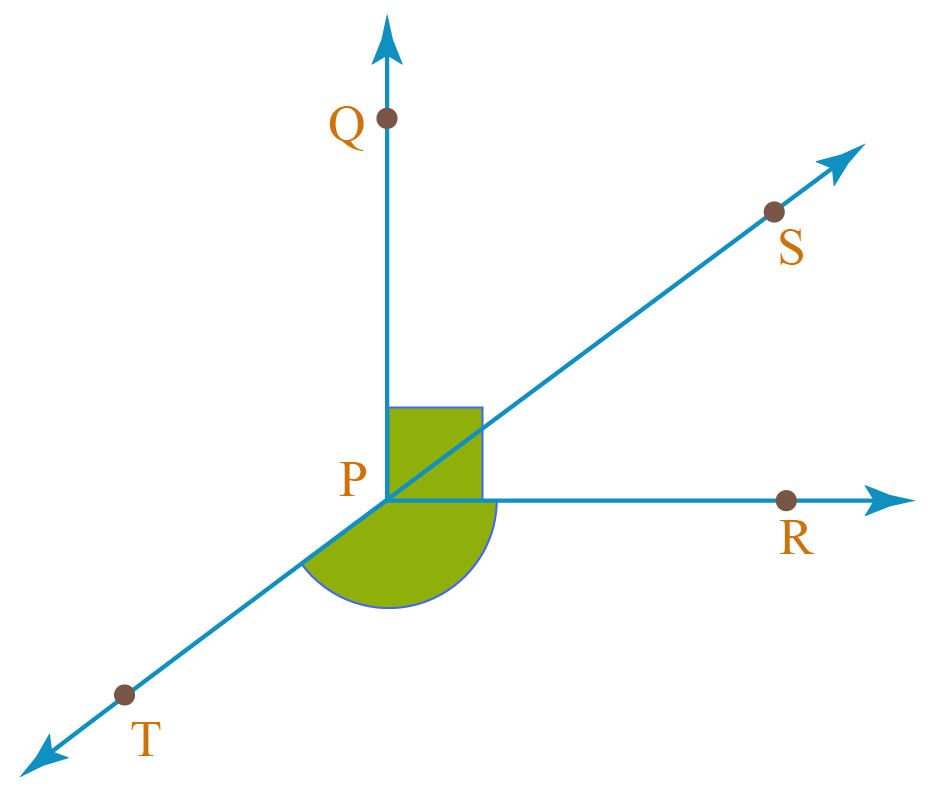CLUEless in Math? Check out how CUEMATH Teachers will explain Complementary Angles to your kid using interactive simulations & worksheets so they never have to memorise anything in Math again!

Explore Cuemath Live, Interactive & Personalised Online Classes to make your kid a Math Expert. Book a FREE trial class today!

## Practice Questions

IMO (International Maths Olympiad) is a competitive exam in Mathematics conducted annually for school students. It encourages children to develop their math solving skills from a competition perspective.

## 1. How do you find a complementary angle?

If the sum of two angles is 90 degrees, then we say that they are complementary.

Thus, the complement of an angle is obtained by subtracting it from 90.

For example, the complement of $$40^\circ$$ is $$90-40=50^\circ$$.

## 2. What is the complementary angle of 40 degrees?

The complement of an angle is obtained by subtracting it from 90 degrees.

Thus, the complement of $$40^\circ$$ is $$90-40=50^\circ$$.

## 3. How do you find the value of x in complementary angles?

If two angles in terms of $$x$$ are given to be complementary, we just set their sum equal to 90 degrees and solve the resultant equation.

You can refer to "Example 2" and "Example 3" under the "Solved Examples" section of this page.

## 4. How do you find the ratio of two complementary angles?

The ratio of two complementary angles is found just like how we find the ratio of any two quantities.

For example, the ratio of the complementary angles $$40^\circ$$ and $$50^\circ$$ is $$\dfrac{40}{50}= \dfrac{4}{5}$$

More Important Topics
Numbers
Algebra
Geometry
Measurement
Money
Data
Trigonometry
Calculus